☆本站外汇EA驻群后全部免费下载！请点击加入QQ下载群：1128719745 ，由于免费EA无法尽数测试，请自测和做好风控！      >>>原云狐网

# 第三代神经网络：深度网络 EA，外汇EA测评，外汇EA网站大全

### 1. 第二代神经网络

• 函数逼近的一组点（回归）；
• 根据指定类集进行数据分类；
• 识别预先未知的原型类的数据聚类；
• 信息压缩；
• 恢复丢失的数据；
• 联想记忆；
• 优化，最优控制等。

1.1. 连结的体系结构

Hopfield网络（相联存贮器，数据聚类，优化）

1.2. 神经网络的主要类型

1.2.1. 多层全连接的前馈网络MLP（多层感知器）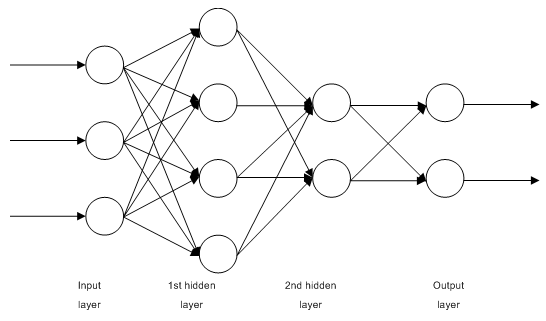1.2.2. Jordan网络是典型的循环网络并且和Elman网络类似。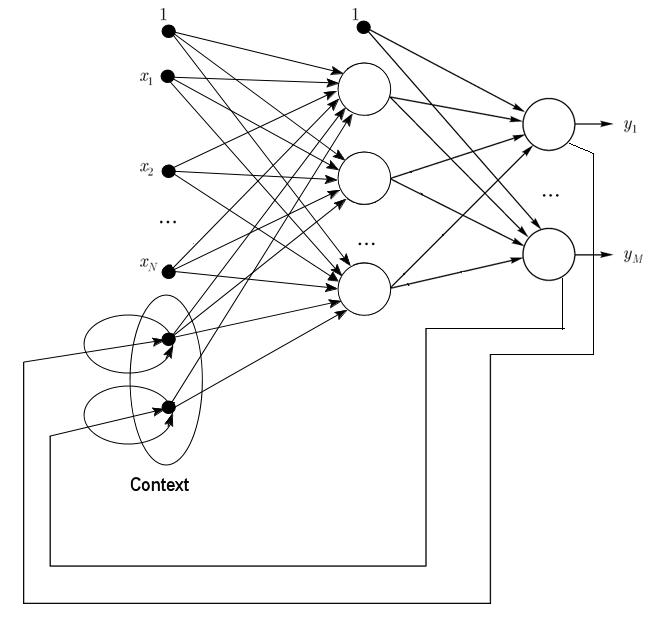1.2.3. Elman网络是典型的循环网络并且和Jordan网络类似。Elman和Jordan网络的区别在于在Elman网络中承接层神经元的输入由输出神经元而不是隐含神经元决定。另外，在承接层神经元中无直接反馈。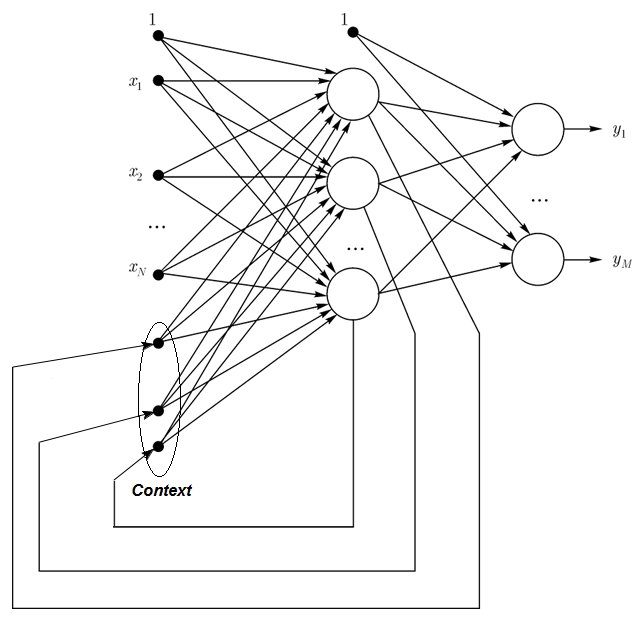1.2.4. 径向基函数网络（RBF）- 是一个前馈神经网络，包含径向对称神经元的一个中间（隐藏）层。这样的神经元通过某些非线性Gaussian算法将其同特定输入向量间的距离转换为中心距。

RBF网络相比多层前馈网络而言有很多优点。首先，它们模仿（不确定这个词是否恰当）仅有一个中间层的任意一个非线性函数，省得开发者决定层数。然后，输出层线性组合参数就能够使用众多广为人知的线性优化算法来进行优化了。后者运行很快并且没有会极大干扰反向传播的本地极小值。这也是为何当使用反向传播时RBF网络的学习速度要块很多的原因。

RBF的缺点：这些网络具有弱外推特性并且当输入向量非常大时会变的低效。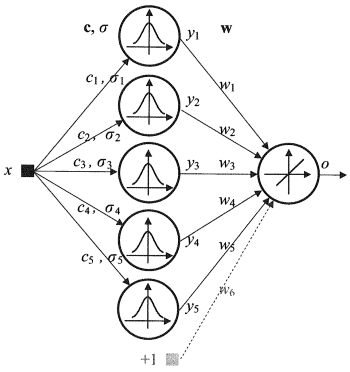1.2.5. 动态学习矢量量化，DLVQ 网络同自组织图（SOM）非常类似。不像SOM，DLVO能够进行有监督的学习并且和原型之间没有依赖关系。矢量量化比聚类的用途更为广泛。

1.2.6. Hopfield网络在执行过程中，这种网络动态收敛到平衡状态中的一种。这些平衡状态是称为网络能量的本地功能极小值。这样的网络可以用作一个内容寻址关联存储器系统、一个过滤器以及解决一些优化问题。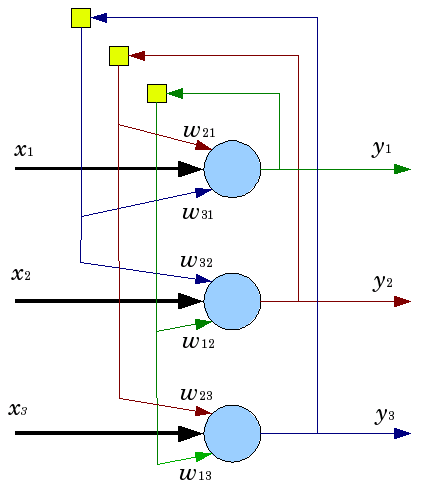1.3. 训练方法

1.3.1. 神经网络学习的主要规则

1.3.1.1. 误差纠正

1.3.1.2. Boltzmann 规则

Boltzmann法则是一种混沌学习算法，它类比热力学理论中的原则。它根据期望的概率分布来调整神经元权重系数。Boltzmann学习算法可以被认为是误差纠正算法的一个特例，它的误差是指两个模型中状态之间的相关性差异。

1.3.1.3. Hebb 规则

Hebb算法是最著名的神经网络学习算法。这个算法的思想是，如果一个突触的两侧的神经元同时并定期时常激活，那么触突连结的强度增加。此处一个重要的特性是，触突的权重仅当其连结的神经元激活时才改变。有很多此算法的变种，区别在于触突权重修改的方式。

1.3.1.4. 竞争性学习1.4. 缺点

• 利用神经网络的主要困难是所谓的“维数灾难”。当输入维度及层数增加后，网络的复杂度和学习时间将呈指数级增长并且接收到的结果并非总是最优的。
• 使用神经网络的另一个困难在于传统的神经网络无法解释它们如何解决问题。在某些医药应用领域对此的解释比结果本身更重要。除了最简单的我们不感兴趣的情况外，内部结果表示是机器复杂的，几乎是不可能进行分析的。

### 2. 深度学习

2.1. 背景

Hinton'团队的成果令人印象深刻，因为团队在最后时刻才参加比赛。不仅如此，深度学习系统的开发无需与其相关的特定分子领域的知识。深度学习的成功应用是人工智能领域2012年的又一重大成就。

2.2. 自动编码器自动编码器和受限玻尔兹曼机。差异与特点

2.2.1. 自动编码器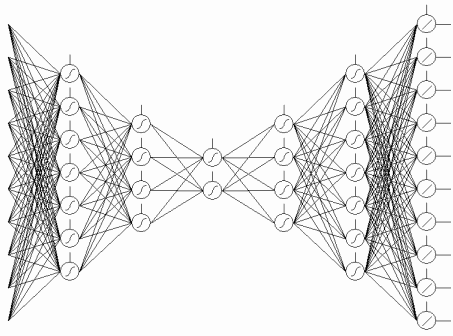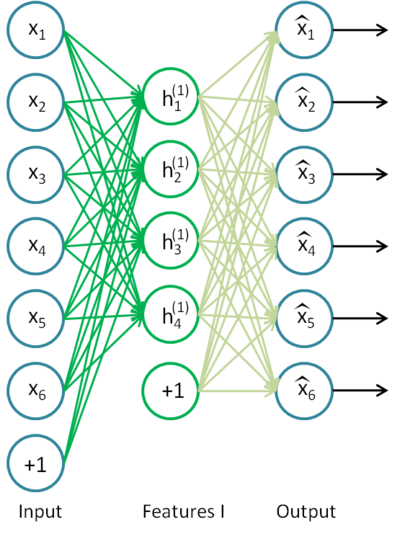2.2.2. 受限玻尔兹曼机，RBM。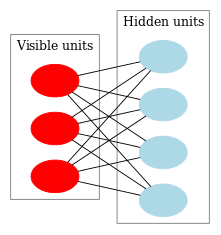CD-k看上去是这样的：

1. 可见神经元的状态被设置为同输入模式一致；
2. 绘制隐含层状态的概率分布；
3. 隐含层的每个神经元被分配状态“1”，意味着同当前状态的分布一致。
4. 可见层状态的概率分布基于隐含层绘制。
5. 如果当前迭代次数少于k，那么返回第二步；
6. 绘制隐含层状态的概率分布；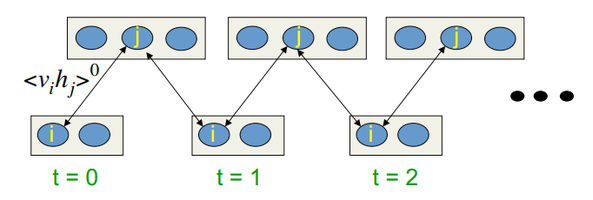2.3. 堆栈式的自动连结网络。堆栈式自动编码器 SAE, 堆栈式自动编码器(堆栈 RBM)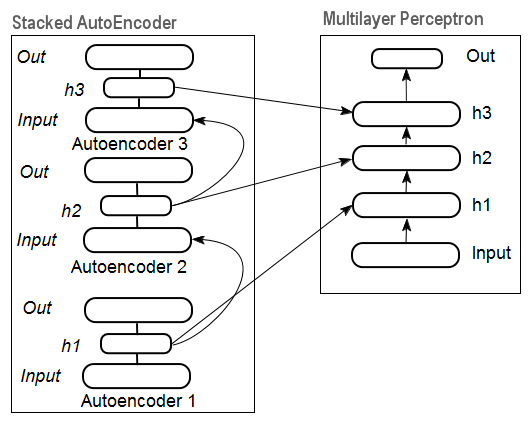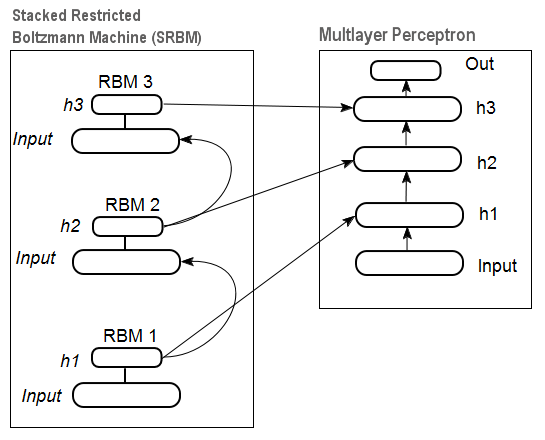2.4. 深度网络训练(DN)。阶段。特点

### 3. 实际实验

3.1. R语言

R是一个GNU开源软件项目，他们基本原则由如下几条：

• 有执行任何目的程序的自由（自由0）；
• 有学习程序如何运行记忆将其修改为己所用的自由（自由1）；
• 有分发程序的副本帮助其他人的自由（自由2）；
• 有改进程序使社会从其改进中收益的自由。

R的优点：

• 如今R在统计计算领域是标准。
• 它正被全球大学的科研团队开发和支撑中。
• 有大量的数据挖掘一切先进领域的开发包。一个想法的公开和用R开发包实现之间的时间间隔通常不会超过2周。
• 最重要的是，它是完全免费的。一位开源操作系统的著名开发者曾说过：“程序就像性一样 — 最好是免费的”。

3.2. 实现方案和问题

1. "deepnet"是一个实现DN SAE和DN SRBM模型的简单开发包。有监督和无监督学习的输入数据集长度是一样的。这使得分两步实施系统微调的可能性不存在了。一开始就要用于构建和测试模型。

2. "darh" 是一个先进、涵盖面广的DN SRBM模型开发包。其中有一个DN SAE模型但是我没有加载成功。这个包是给经验丰富的用户准备的，它能够创建和调整任意复杂度的模型。它基于Hinton独特的Matlab语言程序。

3. "H2O"是一个用于在巨大数据集上（csv文件格式）训练深度网络（不仅仅是）的广泛开发包。

3.3. 准备用于试验的输入和目标数据

1. 选择输入数据（研究、分析、预处理、评估）；将数据集分为训练集、效验集和测试集（抽样）；
2. 在训练集上得到模型，在效验集上验证；
3. 用测试样本评估模型质量，确定最佳模型参数或者通过特定的手段获得匹配测试集的最佳模型。
4. 模型开始工作。

3.3.1. 源数据

```pr.OHLC <- function (o, h, l, c)
{
#Unite quote vectors into a matrix having previously expanded them
#Indexing of time series of vectors in R starts with 1.
#Direction of indexing is from old to new ones.
price <- cbind(Open = rev(o), High = rev(h), Low = rev(l), Close = rev(c))
Med <- (price[, 2] + price[, 3])/2
CO <- price[, 4] - price[, 1]
#add Med and CO to the matrix
price <- cbind(price, Med, CO)
}
```

```> head(price)
Open    High     Low   Close      Med     CO
[1,] 1.33848 1.33851 1.33824 1.33844 1.338375 -4e-05
[2,] 1.33843 1.33868 1.33842 1.33851 1.338550  8e-05
[3,] 1.33849 1.33862 1.33846 1.33859 1.338540  1e-04
[4,] 1.33858 1.33861 1.33856 1.33859 1.338585  1e-05
[5,] 1.33862 1.33868 1.33855 1.33855 1.338615 -7e-05
[6,] 1.33853 1.33856 1.33846 1.33855 1.338510  2e-05
```

3.3.2. 输入数据（预测器）

```> library(TTR)
```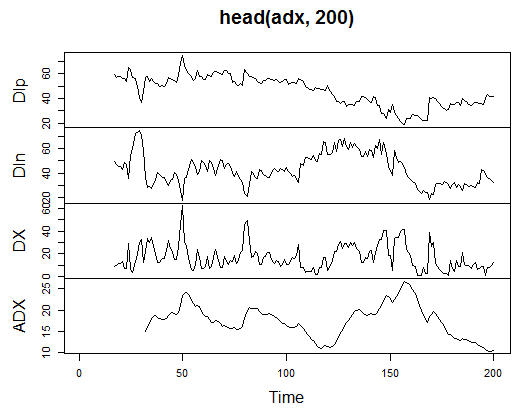```> summary(adx)
Min.   :15.90   Min.   :  5.468   Min.   : 0.00831      Min.   : 5.482
1st Qu.:41.21   1st Qu.: 33.599   1st Qu.: 8.05849      1st Qu.:14.046
Median :47.36   Median : 43.216   Median :16.95423      Median :18.099
Mean   :47.14   Mean   : 46.170   Mean   :19.73032      Mean   :19.609
3rd Qu.:53.31   3rd Qu.: 55.315   3rd Qu.:27.97471      3rd Qu.:23.961
Max.   :80.12   Max.   :199.251   Max.   :81.08751      Max.   :52.413
NA's   :16      NA's   :16        NA's   :16            NA's   :31
```

3.3.2.2. aroon(HL, n) - 1个输出（振荡器）

```> ar<-aroon(price[ , c('High', 'Low')], n = 16)[ ,'oscillator']
> plot(head(ar, 200), t = "l")
> abline(h = 0)
```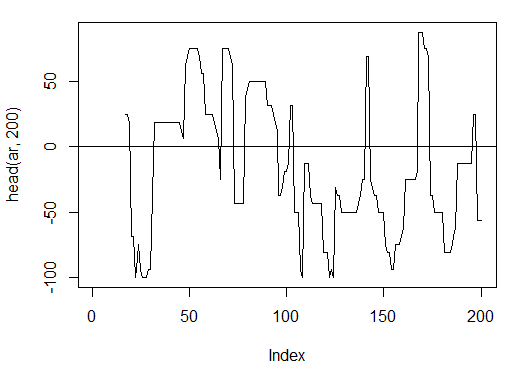```> summary(ar)
Min. 1st Qu.  Median    Mean 3rd Qu.    Max.    NA's
-100.00  -56.25  -18.75   -7.67   43.75  100.00      16
```

3.3.2.3. 商品通道指数 - CCI(HLC, n) - 1个输出

```> cci<-CCI(price[ ,2:4], n = 16)
> abline(h = 0)
```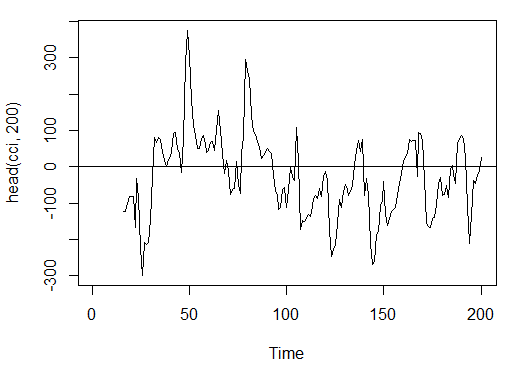```> summary(cci)
Min. 1st Qu.  Median    Mean 3rd Qu.    Max.    NA's
-469.10  -90.95  -18.74  -14.03   66.91  388.20      15
```

3.3.2.4. Chaikin 波动率 - chaikinVolatility (HLC, n) - 1个输出

```> chv<-chaikinVolatility(price[ , 2:4], n = 16)
> summary(chv)
Min.  1st Qu.   Median     Mean  3rd Qu.     Max.     NA's
-0.67570 -0.29940  0.02085  0.12890  0.41580  5.15700       31
> plot(head(chv, 200), t = "l")
> abline(h = 0)
```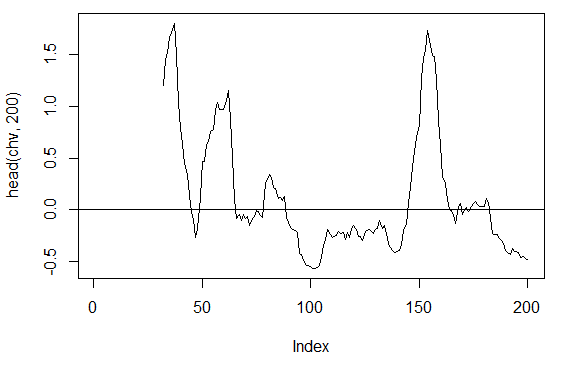3.3.2.5. Chande 动量振荡器 - CMO(Med, n) - 1 out

```> cmo<-CMO(price[ ,'Med'], n = 16)
> plot(head(cmo, 200), t = "l")
> abline(h = 0)
```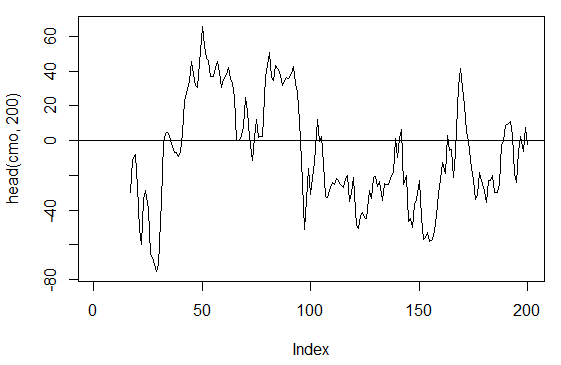```> summary(cmo)
Min.    1st Qu.  Median    Mean 3rd Qu.    Max.    NA's
-97.670 -32.650  -5.400  -6.075  19.530  93.080      16
```

3.3.2.6. MACD 振荡器 - MACD(Med, nFast, nSlow, nSig) 使用1个输出(macd)

```> macd<-MACD(price[ ,'Med'], 12, 26, 9)[ ,'macd']
> plot(head(macd, 200), t = "l")
> abline(h = 0)
```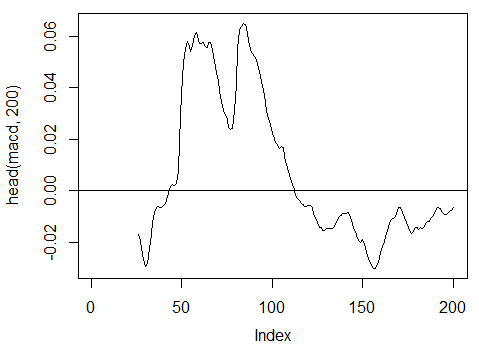```> summary(macd)
Min.   1st Qu.    Median      Mean   3rd Qu.      Max.      NA's
-0.346900 -0.025150 -0.005716 -0.011370  0.013790  0.088880      25
```

3.3.2.7. OsMA(Med,nFast, nSlow, nSig) – 1 out

```> osma<-macd - MACD(price[ ,'Med'],12, 26, 9)[ ,'signal']
> plot(head(osma, 200), t = "l")
> abline(h = 0)
```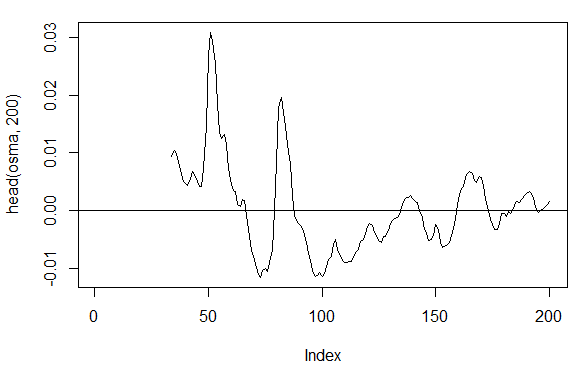```> summary(osma)
Min.  1st Qu.   Median     Mean  3rd Qu.     Max.     NA's
-0.10560 -0.00526  0.00034  0.00007  0.00646  0.05922       33
```

3.3.2.8. 相对强弱指数 - RSI(Med,n) – 1个输出

```> rsi<-RSI(price[ ,'Med'], n = 16)
> plot(head(rsi, 200), t = "l")
> abline(h = 50)
```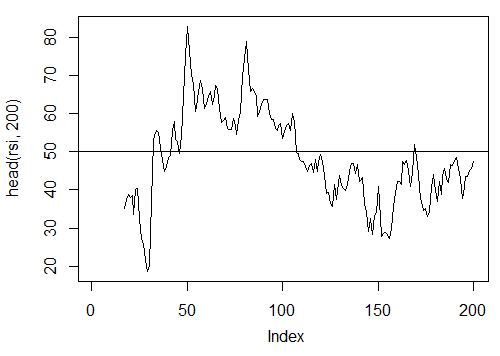```> summary(rsi)
Min. 1st Qu.  Median    Mean 3rd Qu.    Max.    NA's
5.32   37.33   47.15   46.53   55.71   84.82      16
```

3.3.2.9. 随机振荡器 - stoch(HLC, nFastK=14, nFastD=3, nSlowD=3) - 3个输出

```> stoh<-stoch(price[ ,2:4], 14, 3, 3)
```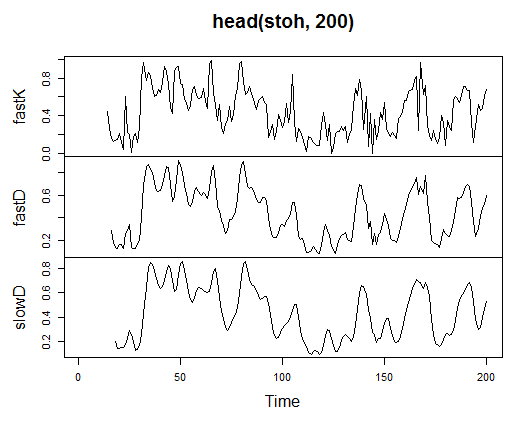```> summary(stoh)
fastK            fastD             slowD
Min.   :0.0000   Min.   :0.01782   Min.   :0.02388
1st Qu.:0.2250   1st Qu.:0.23948   1st Qu.:0.24873
Median :0.4450   Median :0.44205   Median :0.44113
Mean   :0.4622   Mean   :0.46212   Mean   :0.46207
3rd Qu.:0.6842   3rd Qu.:0.67088   3rd Qu.:0.66709
Max.   :1.0000   Max.   :0.99074   Max.   :0.97626
NA's   :13       NA's   :15        NA's   :17
```

3.3.2.10. 随机动量指数 - SMI(HLC, n = 13, nFast = 2, nSlow = 25, nSig = 9) — 2个输出

```> smi<-SMI(price[ ,2:4],n = 13, nFast = 2, nSlow = 25, nSig = 9)
```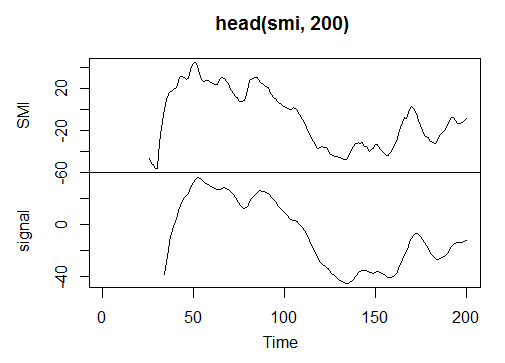```> summary(smi)
SMI              signal
Min.   :-82.185   Min.   :-78.470
1st Qu.:-33.392   1st Qu.:-31.307
Median : -9.320   Median : -8.839
Mean   : -8.942   Mean   : -8.985
3rd Qu.: 15.664   3rd Qu.: 14.069
Max.   : 71.878   Max.   : 63.865
NA's   :25        NA's   :33
```

3.3.2.11. 波动率(Yang 和 Zhang) - volatility(OHLC, n, calc="yang.zhang", N=96)- 1个输出

```> vol<-volatility(price[ ,1:4],n = 16,calc = "yang.zhang", N =96)
```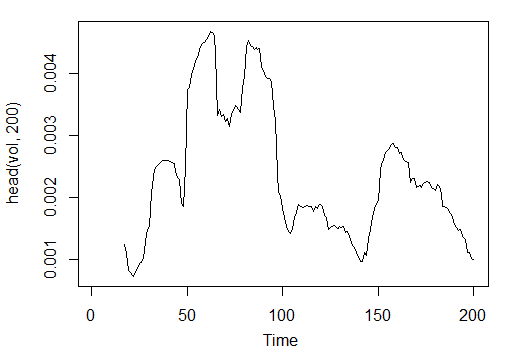```> summary(vol)
Min.  1st Qu.   Median     Mean  3rd Qu.     Max.      NA's
0.000599 0.001858 0.002638 0.003127 0.004015 0.012840      16
```

```In<-function(p = 16){
ar<-aroon(price[ ,c('High', 'Low')], n=p)[ ,'oscillator'];
cci<-CCI(price[ ,2:4], n = p);
chv<-chaikinVolatility(price[ ,2:4], n = p);
cmo<-CMO(price[ ,'Med'], n = p);
macd<-MACD(price[ ,'Med'], 12, 26, 9)[ ,'macd'];
osma<-macd - MACD(price[ ,'Med'],12, 26, 9)[ ,'signal'];
rsi<-RSI(price[ ,'Med'], n = p);
stoh<-stoch(price[ ,2:4],14, 3, 3);
smi<-SMI(price[ ,2:4],n = p, nFast = 2, nSlow = 25, nSig = 9);
vol<-volatility(price[ ,1:4],n = p,calc="yang.zhang", N=96);
In<-cbind(adx, ar, cci, chv, cmo, macd, osma, rsi, stoh, smi, vol);
return(In)
}

> X<-In()
> tail(X)
DIp      DIn       DX      ADX   ar      cci       chv
[3995,] 46.49620 36.32411 12.28212 18.17544 25.0 168.0407 0.1835102
[3996,] 52.99009 31.61164 25.26952 18.61882 37.5 227.7030 0.3189822
[3997,] 58.11948 28.16241 34.72000 19.62515 37.5 145.2337 0.3448520
[3998,] 56.00323 30.48687 29.50206 20.24245 37.5 118.5831 0.3068059
[3999,] 55.96197 28.78737 32.06467 20.98134 37.5 116.5376 0.3517668
[4000,] 54.97777 26.85440 34.36713 21.81795 62.5 160.0767 0.6169701
cmo         macd       osma      rsi     fastK
[3995,] 29.71342 -0.020870825 0.01666593 52.91932 0.8832685
[3996,] 41.89526 -0.009654368 0.02230591 61.49793 0.8833819
[3997,] 30.98237 -0.002051532 0.02392699 58.94513 0.7259475
[3998,] 33.84813  0.003454534 0.02354645 58.00549 0.7930029
[3999,] 38.84892  0.009590136 0.02374564 60.63806 0.8367347
[4000,] 54.71698  0.019303110 0.02676689 66.64815 0.9354120
fastD     slowD        SMI    signal         vol
[3995,] 0.7773581 0.7735064 -35.095406 -47.27712 0.003643196
[3996,] 0.7691688 0.7761507 -26.482951 -43.11828 0.003858942
[3997,] 0.8308660 0.7924643 -19.699762 -38.43458 0.003920541
[3998,] 0.8007775 0.8002707 -13.141932 -33.37605 0.003916109
[3999,] 0.7852284 0.8056239  -6.569699 -28.01478 0.003999789
[4000,] 0.8550499 0.8136852   2.197810 -21.97226 0.004293766
```

3.3.3. 输出数据（目标）

```Out<-function(ch=0.0037){
# ZigZag has values on each bar and not only in the points
zz<-ZigZag(price[ ,'Med'], change = ch, percent = F, retrace = F, lastExtreme = T);
n<-1:length(zz);
# On the last bars substitute the undefined values for the last known ones
for(i in n) { if(is.na(zz[i])) zz[i] = zz[i-1];}
#Define the speed of ZigZag changes and move one bar forward
dz<-c(diff(zz), NA);
#If the speed >0 - signal = 0(Buy), if <0, signal = 1 (Sell) otherwise NA
sig<-ifelse(dz>0, 0, if else(dz<0, 1, NA));
return(sig);
}
```

```> Y<-Out()
> table(Y)
Y
0    1 1567 2423 ```

3.3.4. 清除数据

```Clearing<-function(x, y){
dt<-cbind(x,y);
n<-ncol(dt)
dt<-na.omit(dt)
return(dt);
}
> dt<-Clearing(X,Y); nrow(dt)
 3957
```

3.3.5. 训练和测试样本的形成

3.3.6. 分类平衡

```Balancing<-function(DT){
#Calculate a table with a number of classes
cl<-table(DT[ ,ncol(DT)]);
#If the divergence is less than 15%, return the initial matrix
if(max(cl)/min(cl)<= 1.15) return(DT)
#Otherwise level by the greater side
DT<-if(max(cl)/min(cl)> 1.15){
upSample(x = DT[ ,-ncol(DT)],y = as.factor(DT[ , ncol(DT)]), yname = "Y")
}
#Convert у (factor) into a number
DT\$Y<-as.numeric(DT\$Y)
#Recode у from 1,2 into 0,1
DT\$Y<-ifelse(DT\$Y == 1, 0, 1)
#Convert dataframe to matrix
DT<-as.matrix(DT)
return(DT);
}
```

```dt.b<-Balancing(dt)
x<-dt.b[ ,-ncol(dt.b)]
y<-dt.b[ , ncol(dt.b)]
```

```> library('rminer')
> t<-holdout(y, ratio = 8/10, mode = "random")
```

3.3.7. 预处理

```> spSign<-preProcess(x[t\$tr, ], method = "spatialSign") > x.tr<-predict(spSign, x[t\$tr, ])
> x.ts<-predict(spSign, x[t\$ts, ])
```

3.4. 构建，训练和测试模型

```sae.dnn.train(x, y, hidden = c(10), activationfun = "sigm", learningrate = 0.8, momentum = 0.5, learningrate_scale = 1, output = "sigm", sae_output = "linear",  numepochs = 3, batchsize = 100, hidden_dropout = 0, visible_dropout = 0)
```

• х是输入数据矩阵；
• y 是目标变量的一个向量或者矩阵；
• hidden 是一个各隐含层神经元数量的向量。默认 с(10)；
• activationfun 是一个激活隐含神经元的函数。可以是 "sigm", "linear", "tanh"。默认为 "sigm"；
• learningrate 是梯度下降的训练级数。默认 = 0.8；
• momentum 是一个梯度下降动量。默认 = 0.5；
• learningrate_scale 训练级别在每次迭代后乘以此值。默认 =1.0；
• numepochs 是训练迭代次数。默认 =3；
• batchsize 是被训练的少量数据的规模。默认 =100；
• output 是输出神经元激活函数，可以是"sigm", "linear", "softmax"。默认为 "sigm"；
• sae_output 是SAE输出神经元的激活函数，可以是"sigm", "linear", "softmax"。默认为 "linear"；
• hidden_dropout 是隐含层可删除部分。默认 =0；
• visible_dropout 是可见（输入）层可删除部分。默认 =0。

```> system.time(SAE<-sae.dnn.train(x= x.tr, y= y[t\$tr], hidden=c(100,100,100), activationfun = "tanh", learningrate = 0.6, momentum = 0.5, learningrate_scale = 1.0, output = "sigm", sae_output = "linear", numepochs = 10, batchsize = 100, hidden_dropout = 0, visible_dropout = 0))

training layer 1 autoencoder ...
training layer 2 autoencoder ...
training layer 3 autoencoder ...
sae 训练完毕。

user  system elapsed
12.92    0.00   13.09 ```

```> pr.sae<-nn.predict(SAE, x.ts);
> summary(pr.sae)
V1
Min.   :0.2649   1st Qu.:0.2649
Median :0.5881
Mean   :0.5116   3rd Qu.:0.7410
Max.   :0.7410 ```

```> pr<-ifelse(pr.sae>mean(pr.sae), 1, 0)
> confusionMatrix(y[t\$ts], pr)

Reference
Prediction   0   1
0 316 128
1 134 378

Accuracy : 0.7259
95% CI : (0.6965, 0.754)
No Information Rate : 0.5293
P-Value [Acc > NIR] : <2e-16

Kappa : 0.4496
Mcnemar's Test P-Value : 0.7574

Sensitivity : 0.7022
Specificity : 0.7470
Pos Pred Value : 0.7117
Neg Pred Value : 0.7383
Prevalence : 0.4707
Detection Rate : 0.3305
Detection Prevalence : 0.4644
Balanced Accuracy : 0.7246

'Positive' Class : 0
```

```> new.x<-predict(spSign,tail(dt[ ,-ncol(dt)], 500))
> pr.sae1<-nn.predict(SAE, new.x)
> pr.sig<-ifelse(pr.sae1>mean(pr.sae1), -1, 1)
> table(pr.sig)
pr.sig
-1   1
235 265
> new.y<-ifelse(tail(dt[  , ncol(dt)], 500) == 0, 1, -1)
> table(new.y)
new.y
-1   1
201 299
> cm1<-confusionMatrix(new.y, pr.sig)
> cm1

Reference
Prediction  -1   1
-1 160  41
1   75 224

Accuracy : 0.768
95% CI : (0.7285, 0.8043)
No Information Rate : 0.53
P-Value [Acc > NIR] : < 2.2e-16

Kappa : 0.5305
Mcnemar's Test P-Value : 0.002184

Sensitivity : 0.6809
Specificity : 0.8453
Pos Pred Value : 0.7960
Neg Pred Value : 0.7492
Prevalence : 0.4700
Detection Rate : 0.3200
Detection Prevalence : 0.4020
Balanced Accuracy : 0.7631

'Positive' Class : -1
```

```> bal<-cumsum(tail(price[ , 'CO'], 500) * pr.sig)
> plot(bal, t = "l")
> abline(h = 0)
```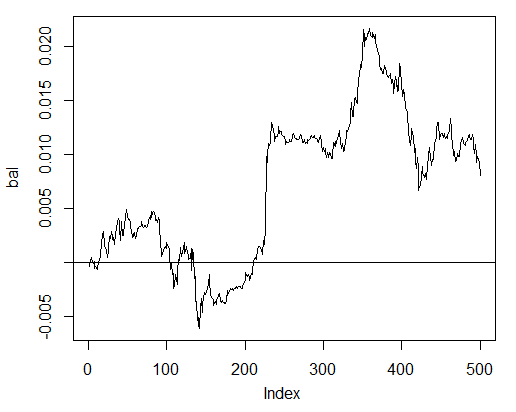```> bal.zz<-cumsum(tail(price[ , 'CO'], 500) * new.y)
> plot(bal.zz,  t = "l")
> lines(bal,  col = 2)
```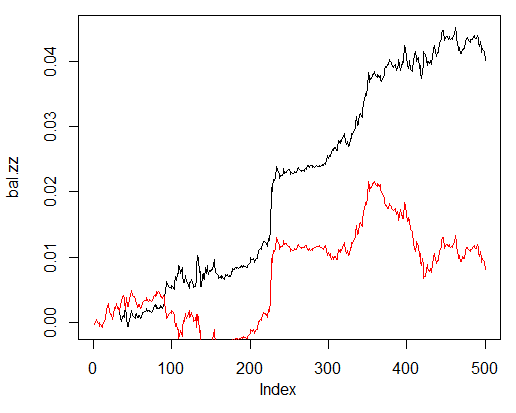```Estimation<-function(X, Y, r = 8/10, m = "random", norm = "spatialSign",
h = c(10), act = "tanh", LR = 0.8, Mom = 0.5,
out = "sigm", sae = "linear", Ep = 10, Bs = 50,
CM=F){
#Indices of the training and test data set
t<-holdout(Y, ratio = r, mode = m)
#Parameters of preprocessing
prepr<-preProcess(X[t\$tr,  ], method = norm)
#Divide into train and test data sets with preprocessing
x.tr<-predict(prepr, X[t\$tr,  ])
x.ts<-predict(prepr, X[t\$ts,  ])
y.tr<- Y[t\$tr]; y.ts<- Y[t\$ts]
#Train the model
SAE<-sae.dnn.train(x = x.tr , y = y.tr , hidden = h,
activationfun = act,
learningrate = LR, momentum = Mom,
output = out, sae_output = sae,
numepochs = Ep, batchsize = Bs)
#Obtain a forecast on the test data set
pr.sae<-nn.predict(SAE, x.ts)
#Recode it into signals 1,0   pr<-ifelse(pr.sae>mean(pr.sae), 1, 0)
#Calculate the Accuracy coefficient or classification error
if(CM) err<-unname(confusionMatrix(y.ts, pr)\$overall)
if(!CM) err<-nn.test(SAE, x.ts, y.ts, mean(pr.sae))
return(err)
}
```

• X – 输入原始预测因子矩阵；
• Y – 目标变量向量；
• r – train/test比例；
• m – 采样模式（随机或连续）；
• norm – 输入参数规范化模式 ([ -1, 1]= "spatialSign";[0, 1]="range")；
• h – 隐含层神经元向量；
• act – 隐含神经元激活函数；
• LR – 训练级数；
• Мом — 动量；
• out – 输出层激活函数；
• sae – 自动编码器激活函数；
• Ep – 训练周期数量；
• Bs – 小样本尺寸；
• СM– 布尔型变量，如果TRUE打印 Accuracy。否则打印 Err。

```> Err<-Estimation(X = dt[ ,-ncol(dt)], Y = dt[ ,ncol(dt)], h=c(30, 30, 30), LR= 0.7)

training layer 1 autoencoder ...
training layer 2 autoencoder ...
training layer 3 autoencoder ...
sae 训练完毕。

> Err
 0.1376263 ```

Testing() 函数通过预测信号或者理想信号(ZigZag)来计算资金曲线：

```Testing<-function(dt1, dt2, r=8/10, m = "random", norm = "spatialSign",
h = c(10), act = "tanh", LR = 0.8, Mom = 0.5,
out = "sigm", sae = "linear", Ep = 10, Bs=50,
pr = T, bar = 500){
X<-dt1[  ,-ncol(dt1)]
Y<-dt1[  ,ncol(dt1)]
t<-holdout(Y,  ratio = r,  mode = m)
prepr<-preProcess(X[t\$tr,  ], method = norm)
x.tr<-predict(prepr, X[t\$tr,  ])
y.tr<- Y[t\$tr];
SAE<-sae.dnn.train(x = x.tr , y = y.tr , hidden = h,
activationfun = act,
learningrate = LR, momentum = Mom,
output = out, sae_output = sae,
numepochs = Ep, batchsize = Bs)
X<-dt2[ ,-ncol(dt2)]
Y<-dt2[ ,ncol(dt2)]
x.ts<-predict(prepr, tail(X, bar))
y.ts<-tail(Y, bar)
pr.sae<-nn.predict(SAE, x.ts)
sig<-ifelse(pr.sae>mean(pr.sae), -1, 1)
sig.zz<-ifelse(y.ts == 0, 1,-1 )
bal<-cumsum(tail(price[  ,'CO'], bar) * sig)
bal.zz<-cumsum(tail(price[  ,'CO'], bar) * sig.zz)
if(pr) return(bal)
if(!pr) return(bal.zz)
}
```

• dt1 – 用于训练网络的输入矩阵和目标变量；
• dt2 - 用于测试网络的输入矩阵和目标变量；
• pr – Boolean 变量， 如果为TRUE根据预测信号交易打印资金曲线，否则根据ZigZag指标交易打印资金曲线；
• bar - 用于计算资金曲线的柱形数量。

```> Bal<-Testing(dt.b, dt, h=c(30, 30, 30), LR= 0.7)

training layer 1 autoencoder ...
training layer 2 autoencoder ...
training layer 3 autoencoder ...
sae 训练完毕。

> plot(Bal, t = "l")
> abline(h = 0)
```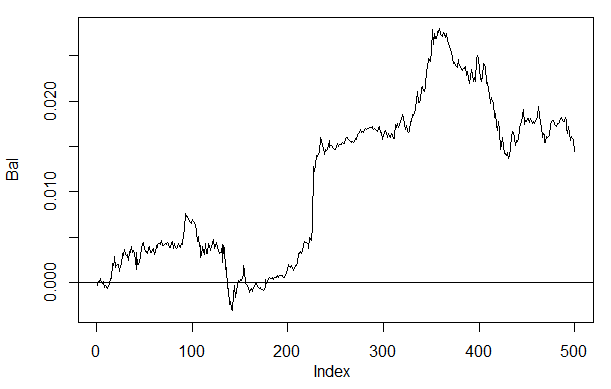```> plot(tail(price[  ,'Close'], 500), t = "l")
> abline(v = c(150,350), col=2)
```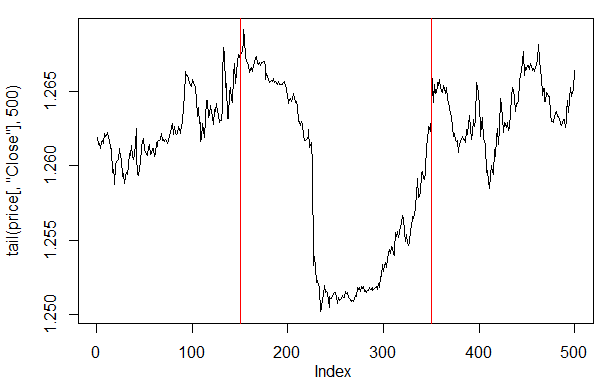```Testing.1<-function(dt1, dt2, r = 8/10, m = "random", norm = "spatialSign",
h = c(10), act = "tanh", LR = 0.8, Mom = 0.5,
out = "sigm", sae = "linear", Ep = 10, Bs = 50,
pr = T, bar = 500, dec=1){
X<-dt1[ ,-ncol(dt1)]
Y<-dt1[ ,ncol(dt1)]
t<-holdout(Y, ratio = r, mode = m)
prepr<-preProcess(X[t\$tr, ], method = norm)
x.tr<-predict(prepr, X[t\$tr, ])
y.tr<- Y[t\$tr];
SAE<-sae.dnn.train(x = x.tr , y = y.tr , hidden = h,
activationfun = act,
learningrate = LR, momentum = Mom,
output = out, sae_output = sae,
numepochs = Ep, batchsize = Bs)
X<-dt2[ ,-ncol(dt2)]
Y<-dt2[ ,ncol(dt2)]
x.ts<-predict(prepr, tail(X, bar))
y.ts<-tail(Y, bar)
pr.sae<-nn.predict(SAE, x.ts)
#Вариант +/- mean
if(dec == 1) sig<-ifelse(pr.sae>mean(pr.sae), -1, 1)
#Вариант 60/40   if(dec == 2) sig<-ifelse(pr.sae>0.6, -1, ifelse(pr.sae<0.4, 1, 0))
sig.zz<-ifelse(y.ts == 0, 1,-1 )
bal<-cumsum(tail(price[  ,'CO'], bar) * sig)
bal.zz<-cumsum(tail(price[  ,'CO'], bar) * sig.zz)
if(pr) return(bal)
if(!pr) return(bal.zz)
}
```

```> set.seed<-1245 > Bal1<-Testing.1(dt.b, dt, h = c(30, 30, 30), LR = 0.7, dec = 1)

training layer 1 autoencoder ...
training layer 2 autoencoder ...
training layer 3 autoencoder ...
sae 训练完毕。

> set.seed<-1245 > Bal2<-Testing.1(dt.b, dt, h = c(30, 30, 30), LR = 0.7, dec = 2)

training layer 1 autoencoder ...
training layer 2 autoencoder ...
training layer 3 autoencoder ...
sae 训练完毕。

> plot(Bal2, t = "l")
> lines(Bal1, col = 2)
```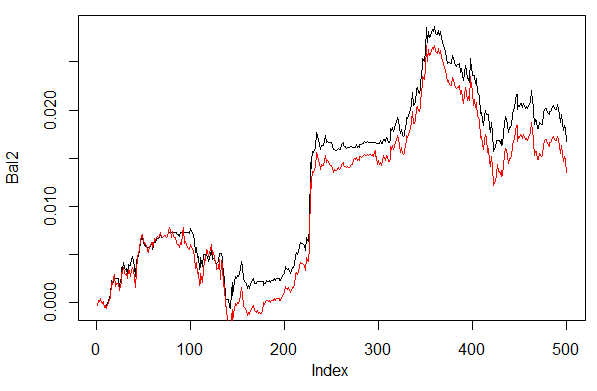3.4.1. 并行计算

```library(doParallel) library(foreach)
puskCluster<-function(){
cores<-detectCores()
cl<-makePSOCKcluster(cores)
registerDoParallel(cl)
clusterSetRNGStream(cl) return(cl)
}
```

```cl<-puskCluster()
stopCluster(cl)
```

```SAE<-foreach(times(ans), .packages = "deepnet") %dopar%
sae.dnn.train(x = x.tr , y = y.tr , hidden = h,
activationfun = act,
learningrate = LR, momentum = Mom,
output = out, sae_output = sae,
numepochs = Ep, batchsize = Bs)
```

times(ans)是我们想要获取的网络编号，.packages指向获取计算函数的包。

`pr.sae<-(foreach(i = 1:ans, .combine = "+") %do%  nn.predict(SAE[[i]], x.ts))/ans`

1. 一个神经网络：

```> system.time(Bal21<-Testing.1(dt.b, dt, h = c(30, 30, 30), LR = 0.7, dec = 2, Ep=300))

training layer 1 autoencoder ...
####loss on step 10000 is : 0.000057 ####loss on step 20000 is : 0.000043 training layer 2 autoencoder ...
####loss on step 10000 is : 0.000081 ####loss on step 20000 is : 0.000086 training layer 3 autoencoder ...
####loss on step 10000 is : 0.000072 ####loss on step 20000 is : 0.000066 sae 训练完毕。

####loss on step 10000 is : 0.069451 ####loss on step 20000 is : 0.079629 深度神经网络训练完毕。
user  system elapsed 115.78    0.00  116.96 > plot(Bal21, t = "l")
> abline(h = 0)
```

2. 3个神经网络的组合：

```> system.time(Bal41<-Testing.2(dt.b, dt, h = c(30, 30, 30), LR = 0.7, Ep=300, dec = 2, ans=3))
user  system elapsed
0.22    0.06  233.64 > lines(Bal41, col=4)

```

3. 4个神经网络组合：

```> system.time(Bal44<-Testing.2(dt.b, dt, h = c(30, 30, 30), LR = 0.7, Ep=300, dec = 2, ans=4))
user  system elapsed
0.13    0.03  247.86 > lines(Bal44, col=2)
```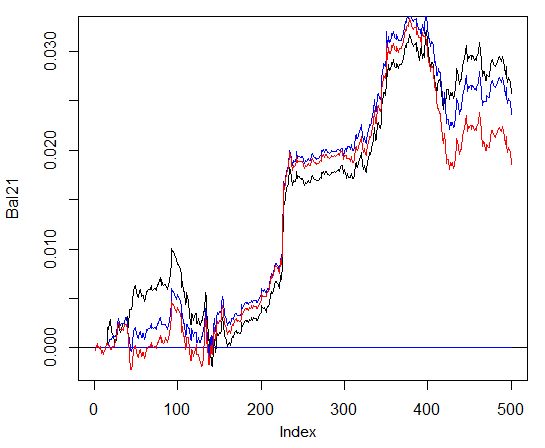```> system.time(Bal0<-Testing.1(dt.b, dt, h = c(30, 30, 30), LR = 0.7, dec = 2, Ep=300, bar=1000))

training layer 1 autoencoder ...
####loss on step 10000 is : 0.000054 ####loss on step 20000 is : 0.000044 training layer 2 autoencoder ...
####loss on step 10000 is : 0.000078 ####loss on step 20000 is : 0.000079 training layer 3 autoencoder ...
####loss on step 10000 is : 0.000090 ####loss on step 20000 is : 0.000072 sae 训练完毕。

####loss on step 10000 is : 0.072633 ####loss on step 20000 is : 0.057917 深度神经网络训练完毕。
user  system elapsed 116.09    0.02  116.26 > max(Bal0)
 0.04725 > plot(Bal0, t="l")
> tail(Bal0,1)
 0.03514 ```

```> system.time(Bal0<-Testing.1(dt.b, dt, h = c(13, 8, 5), LR = 0.7, dec = 2, Ep=300, bar=1000))

training layer 1 autoencoder ...
####loss on step 10000 is : 0.005217 ####loss on step 20000 is : 0.004846 training layer 2 autoencoder ...
####loss on step 10000 is : 0.051324 ####loss on step 20000 is : 0.046230 training layer 3 autoencoder ...
####loss on step 10000 is : 0.023292 ####loss on step 20000 is : 0.026113 sae 训练完毕。

####loss on step 10000 is : 0.057788 ####loss on step 20000 is : 0.056932 深度神经网络训练完毕。
user  system elapsed
64.04    0.01   64.24 报警信息：
In sae\$encoder[[i - 1]]\$W[] %*% t(train_x) + sae\$encoder[[i -  :
longer object length is not a multiple of shorter object length
> lines(Bal0, col="blue")
```

```> system.time(Bal0<-Testing.1(dt.b, dt, h = c(50, 50, 50), LR = 0.7, dec = 2, Ep=300, bar=1000))

training layer 1 autoencoder ...
####loss on step 10000 is : 0.000018 ####loss on step 20000 is : 0.000013 training layer 2 autoencoder ...
####loss on step 10000 is : 0.000062 ####loss on step 20000 is : 0.000048 training layer 3 autoencoder ...
####loss on step 10000 is : 0.000053 ####loss on step 20000 is : 0.000055 sae 训练完毕。

####loss on step 10000 is : 0.096490 ####loss on step 20000 is : 0.084860 深度神经网络训练完毕。
user  system elapsed 186.18    0.00  186.39 > lines(Bal0, col="red")
> max(Bal0)
 0.0543 ```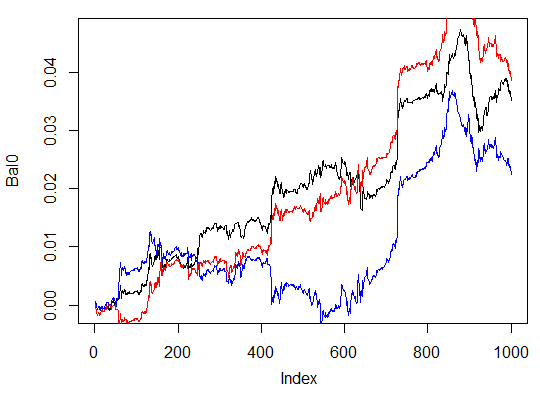### 4. 实现（指标和EA）

• 第一种方式。神经网络的训练在Rstudio中手动执行。在获得可接受的结果后，将网络保存在恰当的目录中。然后在图表上加载EA和指标。EA将加载已训练后的神经网络。指标将每个新柱形上的输入数据组织成向量传入EA。EA呈现神经网络数据，接收交易信号然后执行交易。EA执行常规操作如开仓、平仓，追踪止损等。指标的目的是给EA准备和传递每个新柱形的输入数据，最重要的是，在图表上呈现网络的预测信号。实践证明，可视化控制是评估一个神经网络的最有效的方法。
• 第二种方式。在图表上加载EA和指标。刚加载时，指标传递给EA一个预先准备好的输入输出大数据集。EA开始训练、测试及选择最佳神经网络。这之后的运作和第一种方式一样。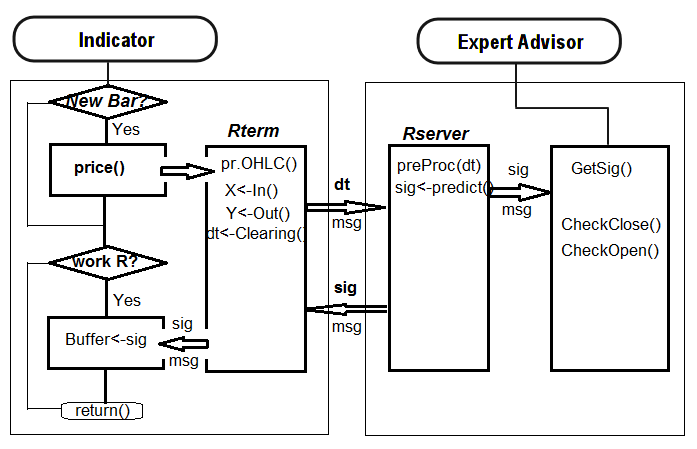4.1. 训练和保存模型

```> dt.b<-Balancing(dt)
> table(dt.b[ ,ncol(dt.b)])
0    1 2288 2288 ```

```> system.time(bal<-Testing.1(dt.b, dt, h = c(50, 50, 50), LR = 0.7, dec = 2, Ep=500, bar=500))

training layer 1 autoencoder ...
####loss on step 10000 is : 0.000017 ####loss on step 20000 is : 0.000015 ####loss on step 30000 is : 0.000015 training layer 2 autoencoder ...
####loss on step 10000 is : 0.000044 ####loss on step 20000 is : 0.000041 ####loss on step 30000 is : 0.000039 training layer 3 autoencoder ...
####loss on step 10000 is : 0.000042 ####loss on step 20000 is : 0.000042 ####loss on step 30000 is : 0.000036 sae 训练完毕。

####loss on step 10000 is : 0.089417 ####loss on step 20000 is : 0.043276 ####loss on step 30000 is : 0.069399 深度神经网络训练完毕。
user  system elapsed 267.59    0.08  269.37 > plot(bal, t="l")
```

```> SAE1<-SAE
> system.time(bal<-Testing.1(dt.b, dt, h = c(50, 50, 50), LR = 0.7, dec = 2, Ep=300, bar=500))

training layer 1 autoencoder ...
####loss on step 10000 is : 0.000020 ####loss on step 20000 is : 0.000016 training layer 2 autoencoder ...
####loss on step 10000 is : 0.000050 ####loss on step 20000 is : 0.000050 training layer 3 autoencoder ...
####loss on step 10000 is : 0.000051 ####loss on step 20000 is : 0.000043 sae 训练完毕。

####loss on step 10000 is : 0.083888 ####loss on step 20000 is : 0.083941 深度神经网络训练完毕。
user  system elapsed 155.32    0.02  156.25 > lines(bal, col=2)
```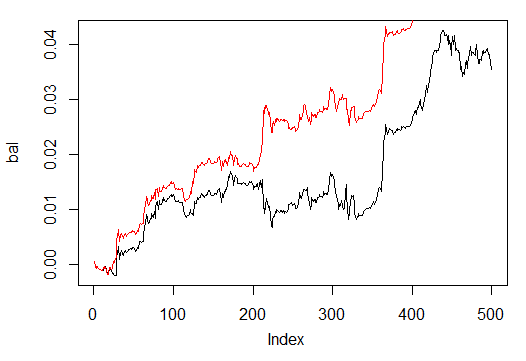`save(SAE, prepr, file="SAE.model")`

```sig.zz<-ifelse(tail(dt[  , ncol(dt)], 500) == 0, 1, -1)
bal.zz<-cumsum(tail(price[  , 'CO'], 500) * sig.zz)
Kzz<-mean(bal.zz / bal)
> Kzz
 0.9173312
```

```> plot(bal/bal.zz, t="l")
> summary(bal/bal.zz)
Min.  1st Qu.   Median     Mean  3rd Qu.     Max.
-15.2500   0.7341   0.7844   0.9173   0.8833  55.0000 ```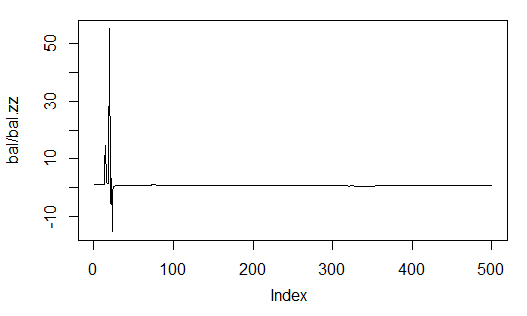```> Kb<-tail(bal,1)/length(bal)*10^Dig
> Kb
 11.508 ```

ZigZag信号交易：

```> Kbz<-tail(bal.zz,1)/length(bal)*10^Dig
> Kbz
 13.784 ```

EA将在图表上显示下述参数：OP - 执行操作，Acc - 准确率，K - 是之前定义的Kb，Kmax - 同Kb一样，但是由资金高点定义，给出这个参数的最大值和最近柱形上的值的差异。

4.2. 安装和加载命令

1. i_SAE.mq4 指标，放在 ~/MQL4/Indicators/ 目录下。
2. e_SAE.mq4 EA，放在 ~/MQL4/Experts/ 目录下。
3. mt4Rb7.dll 库，放在 ~/MQL4/Libraries/ 目录下。
4. mt4Rb7.mqh 头文件，放在 ~/MQL4/Include/ 目录下。库和头文件由Bernd Kreuss开发。文件名称包含了最新更新版本的索引号(b7)。当有众多同名版本时会让人感到困惑，要花很多时间来理清。
5. R的脚本：i_SAE.r（主要指标脚本），i_SAE_fun.r（指标脚本函数），e_SAE.r（EA脚本），e_SAE_init.r（EA初始化脚本）， SAE_SetDir.r（用于验证的脚本以及创建目录的脚本）。脚本既不依赖于货币对也不依赖时间框架，它们能够存放在一个独立的目录中。我是放在 "C:Rdata/SAE/" 下。"C:Rdata/" 目录包含不同的脚本，它们不附属于任何一个项目。如果你的脚本所在目录和我的不一样，那么亲保证在指标和EA中修改使得其能够正确的调用脚本。
6. SAE.model 是含有"SAE"模型及规范化参数"prepr"的文件。模型在EURUSD (M30)上训练所得，最新的行情时间是 14.10.2014。训练过程上面已经阐释。

4.3. 改进质量特征的途径和方法

1. 改变用作输入的指标集。
2. 改变输入数据规范化的方式。
3. 优化“监督者”和指标的输入参数。
4. 为具有两列的矩阵改变输入变量的编码。校准预测信号。
5. 优化网络参数（隐含层的神经元数量，层数，学习级数，周期数）。

### 总结

`【下载必读】如何下载本站资源：*本站资源全部免费下载，请加QQ群：1128719745,验证消息【EAMT】，通过后在【群文件】搜索名称下载！群内禁发广告，每个QQ仅1次申请资格，请珍惜！*群内资源采用最新的压缩方式，双击直接解压，解压缩时请关闭杀毒软件或允许。无毒请放心使用！`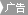★据此入市，风险自担，谨防损失！★